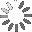# Example sentences for: plotinput

How can you use “plotinput” in a sentence? Here are some example sentences to help you improve your vocabulary:

• deconvolution:table([usedata = 3,fitfunc = 2,plotinput = 1,Nexp = 3,breakpoint = 5, scale_smooth= 1]);

• The parameter deconvolution is a Maple table characterizing the type of deconvolution calculation: usedata determines whether the bolus response function, the input function, or both functions are found, fitfunc determines the deconvolution technique that is used, plotinput specifies the graphical output, Nexp is the number of exponentials in the bolus response function, breakpoint is either the type of procedure used to determine the breakpoints for the spline deconvolution, or the time interval for the uniform deconvolution and scale_smooth scales the smoothing parameter.

## Search

Search for example sentencesLoading...